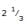# Races and Games

## 12 Questions MCQ Test General Knowledge | Races and Games

Description
Attempt Races and Games | 12 questions in 10 minutes | Mock test for Current Affairs preparation | Free important questions MCQ to study General Knowledge for Current Affairs Exam | Download free PDF with solutions
QUESTION: 1

Solution:
QUESTION: 2

Solution:
QUESTION: 3

### In a 300 m race A beats B by 22.5 m or 6 seconds. B's time over the course is:

Solution:
QUESTION: 4
A and B take part in 100 m race. A runs at 5 kmph. A gives B a start of 8 m and still beats him by 8 seconds. The speed of B is:
Solution:
QUESTION: 5
A can run 224 metre in 28 seconds and B in 32 seconds. By what distance A beat B?
Solution:
QUESTION: 6
10. A and B take part in 100 m race. A runs at 5 kmph. A gives B a start of 8 m and still beats him by 8 seconds. The speed of B is:
Solution:
QUESTION: 7

A istimes as fast as B. If A gives B a start of 80m,how long should the race course be so that both of them reach at the same time?

Solution:
QUESTION: 8
In a 500 m race, the ratio of the speeds of two contestants A and B is 3 : 4. A has a start of 140 m. Then, A wins by: B.
Solution:
QUESTION: 9
In a 100 m race, A can give B 10 m and C 28 m. In the same race B can give C :
Solution:
QUESTION: 10

At a game of billiards, A can give B 15 points in 60 and A can give C to 20 points in 60. How many points can B give C in a game of 90?

Solution:
QUESTION: 11
In a race of 200 m, A can beat B by 31 m and C by 18 m. In a race of 350 m, C will beat B by:
Solution:
QUESTION: 12
In a 100 m race, A can beat B by 25 m and B can beat C by 4 m. In the same race, A can beat C by:
Solution:Use Code STAYHOME200 and get INR 200 additional OFF Use Coupon Code